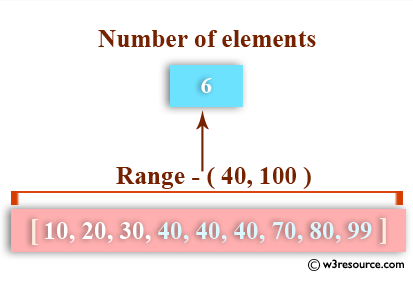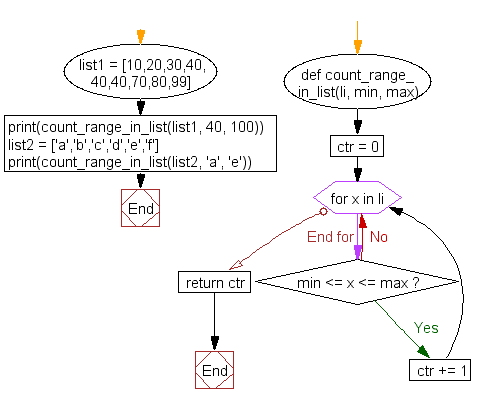﻿ Python: Count the number of elements in a list within a specified range - w3resource# Python: Count the number of elements in a list within a specified range

## Python List: Exercise - 31 with Solution

Write a Python program to count the number of elements in a list within a specified range.Sample Solution:-

Python Code:

``````def count_range_in_list(li, min, max):
ctr = 0
for x in li:
if min <= x <= max:
ctr += 1
return ctr

list1 = [10,20,30,40,40,40,70,80,99]
print(count_range_in_list(list1, 40, 100))

list2 = ['a','b','c','d','e','f']
print(count_range_in_list(list2, 'a', 'e'))
```
```

Sample Output:

```6
5
```

Flowchart:## Visualize Python code execution:

The following tool visualize what the computer is doing step-by-step as it executes the said program:

Python Code Editor:

Have another way to solve this solution? Contribute your code (and comments) through Disqus.

What is the difficulty level of this exercise?

Test your Python skills with w3resource's quiz

﻿

## Python: Tips of the Day

Floor Division:

When we speak of division we normally mean (/) float division operator, this will give a precise result in float format with decimals.

For a rounded integer result there is (//) floor division operator in Python. Floor division will only give integer results that are round numbers.

```print(1000 // 300)
print(1000 / 300)```

Output:

```3
3.3333333333333335```